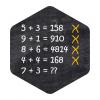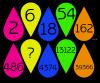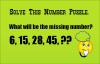BRAIN TEASERSBrain Teasers User Profile

 Calculate 7+3 If 5+3=158, 9+1=910, 8+6=4814, 4+4=168 then 7+3=?Find the pattern to determin... Find the pattern to determine what the missing number is.What will be the missing number? Solve this number puzzle. What will be the missing number? (6, 15, 28, 45, ??)